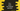# How to print an inverted pyramid in C++## How to print an inverted pyramid in C++:

This post will show you how to print an inverted pyramid in C++. The inverted pyramid looks as like below:

``````* * * * *
* * * *
* * *
* *
*``````

This is an inverted pyramid of height 5. Our program will take the height as input from the user and print that inverted pyramid.

Before writing the program, let’s write down the algorithm for printing the inverted pyramid.

### Algorithm:

Let’s take a look at the below pyramid:

``````* * * * *
y* * * *
yy* * *
yyy* *
yyyy*``````

It is similar to the above one. The only difference is that we are using y for this program but blank space for the above one. If we write the algorithm for this pyramid, we can write the algorithm for the above algorithm easily.

If we consider h as the height of the triangle and i as the currect row, which starts from 0 and ends at 4,

• The first-row, i.e. for i = 0, it prints height number of * with a blank space in between
• The second-row prints 1 number of y and height - 1 number of * with blank space in between the *.
• The third-row prints 2 number of y and height - 2 number of * with blank space in between the *.

So, for row i, it prints i number of y and height - i number of *.

Based on that, we can write the algorithm as below:

• Start one for loop from i = 0 to i = height - 1.
• For each iteration of the loop, print blank for i number of times and print * for height - i number of times with a space in between.
• At the end of each iteration print a new line.

### C++ program:

Below is the complete C++ program :

``````#include <iostream>
using namespace std;

int main()
{
int height;
cout << "Enter the height of the pyramid : " << endl;
cin >> height;

for (int i = 0; i < height; i++)
{
for (int j = 0; j < i; j++)
{
cout << " ";
}
for (int j = 0; j < height - i; j++)
{
cout << "* ";
}
cout << endl;
}
}``````

It will print output as like below:

``````Enter the height of the pyramid :
10
* * * * * * * * * *
* * * * * * * * *
* * * * * * * *
* * * * * * *
* * * * * *
* * * * *
* * * *
* * *
* *
* ``````

We can give any height and it will print that pyramids.